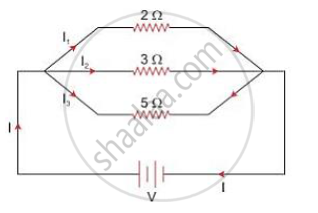# You Have Three Resistors of Values 2ω, 3ω and 5ω. How Will You Join Them So that the Total Resistance is Less than 1ω? Draw Diagram and Find the Total Resistance. - Physics

You have three resistors of values 2Ω, 3Ω and 5Ω. How will you join them so that the total  resistance is less than 1Ω? Draw diagram and find the total resistance.

#### Solution

The three resistors should be connected in parallel
To get a total resistance less than 1ΩLet ' R be the total resistance.
Then,

1/R =1/2+1/3+1/5=(15+10+16)/30=31/30

Or, R =30/31=0.97Ω

Concept: System of Resistors - Resistances in Parallel
Is there an error in this question or solution?

#### APPEARS IN

Selina Concise Physics Class 10 ICSE
Chapter 8 Current Electricity
Exercise 8 (B) | Q 8 | Page 201